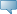Catalog Entries

Fall 2021
Jan 21, 2022Select the link beside Class Schedule to find available classes for the course.

 MATH 4370 - Linear Algebra and Matrix Analysis Vector and matrix norms, matrix factorizations, eigenvalues and eigenvectors, theory of non-negative matrices. Applications to differential equations, math biology, numerical analysis, digital image processing, data mining, GPS, Markov chains, graph theory, etc. will be given in this course. Not to be held with the former MATH 4310. Prerequisite: MATH 2090 or MATH 2091 or the former MATH 2300 (B) or the former MATH 2301 (B) or the former MATH 2350 or the former MATH 2352. - 3.0 Credit hours Science Mathematics Department Course Attributes: Mathematics Requirement, Science Requirement for BA, Science